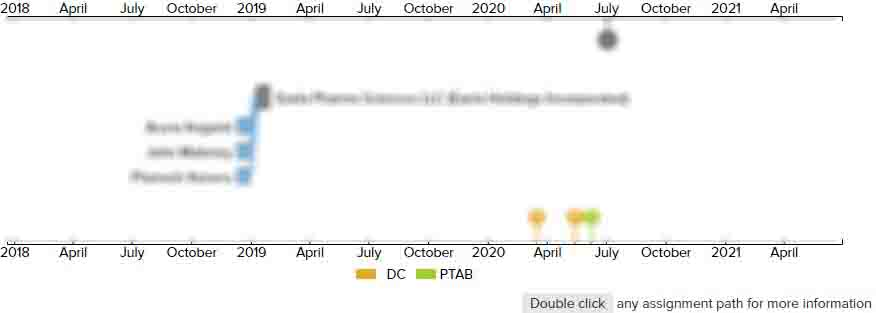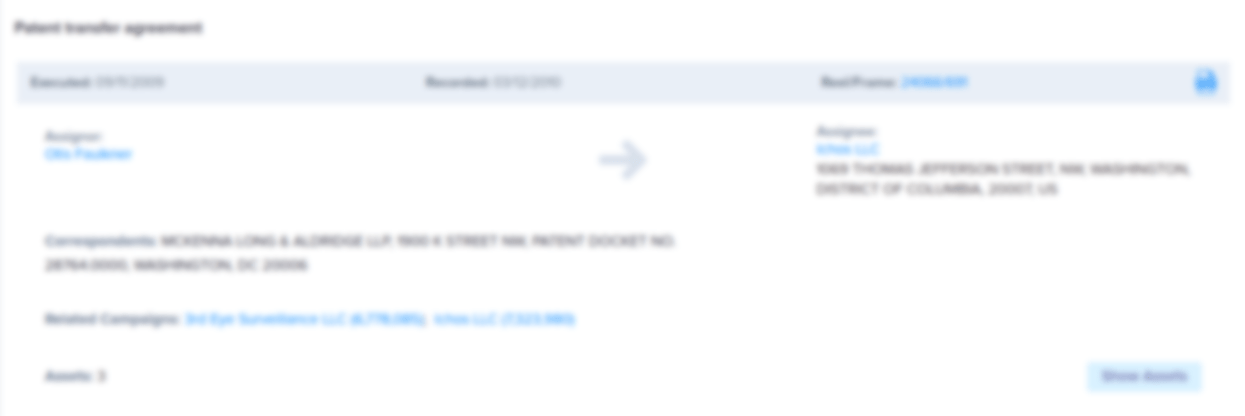# Channel interleaving method and apparatus

• US 11,082,070 B2
• Filed: 12/27/2019
• Issued: 08/03/2021
• Est. Priority Date: 06/27/2017
• Status: Active Grant
##### First Claim
Patent Images

1. A channel interleaving method of a polar (Polar) code, the method being performed by a receive end device, wherein the receive end device comprising a processing apparatus configured to perform the method, and the method comprising:

• determining an M_r-row and M_c-column matrix used for interleaving, whereincolumn sequence numbers of the matrix are represented by;

[0, 1, . . . , ic, . . . , M_c

1], wherein ic is greater than or equal to 0, and is less than or equal to M_c

1; and

row sequence numbers of the matrix are represented by;

[0, 1, . . . , ir, . . . , M_r

1], wherein ir is greater than or equal to 0, and is less than or equal to M_r

1;

writing to-be-interleaved bits into the matrix; and

permutating, based on permutation patterns of the column sequence numbers and/or permutation patterns of the row sequence numbers, the matrix into which the to-be-interleaved bits are written, whereinthe permutation patterns of the column sequence numbers are represented by;

[Pc(0), Pc(1), . . . , Pc(ic), . . . , Pc(M_c

1)], wherein Pc(ic) is obtained by performing pruned bit reverse (PBR, pruned bit reverse))based mapping on column sequence number ic; and

the permutation patterns of the row sequence numbers are represented by;

[Pr(0), Pr(1), . . . , Pr(ir), . . . , Pr(M_r

1)], wherein Pr(ir) is obtained by performing pruned bit reverse (PBR, pruned bit reverse)—

based mapping on row sequence number ir; and

reading interleaved bits from the permutated matrix.

• ##### 2 Assignments
Timeline View
Assignment View×
×